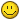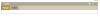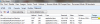## Recommended Posts

Hi,

In my app, fusion charts are not getting loaded for the first time after clearing browser cache.For example if I try to load a Line chart, for the first time it will not come , and next time it will load.Same for all type if charts.In other words if I enable the IE setting : 'Always refresh from server' the charts are not getting loaded at all.May be because the swf is not available in the cache.But it should get loaded when the chart is rendered rt? if IE setting : 'Always refresh from server' is disabled ,when tried for the second time it gets loaded.I am loading the charts using the 'updateFusionCharts' jquery method.What can be the reason for this.Pls help

Fusioncharts version : xt 3.2.2 .

IE version : 9

Deepti.

Edited by deepti

##### Share on other sites

The issue is not there in case of JS rendering.So it seems to be an issue in loading the swf file.It will be great if someone can give me a reply whether this is a known issue or if there is a work around for this.

##### Share on other sites

Hi,

Can some one please give a reply for this as I am not yet able to find a solution for this ?

Thanks,

Deepti

##### Share on other sitesHi Deepti,

A warm welcome to FusionCharts Forum!Apologies for the delayed response.

Can you please provide some information on following points?

> Send us the chart XML and sample code as well.

> What chart type are you using?

> Also send us the screen-shot of your issue as well.

##### Share on other sites

Thanks for the response :

Fig 1 image shows what I am expecting and what I am getting when cache is not cleared in IE.

But when cache is cleared in IE I am getting it as shown in Fig 2.If you see the chart is not rendered.

The request and response flow in IE is as in Fig 3.

I am using the JS code shown below to render the chart. Where compId is the id of the div which holds the chart.

function renderChart(ajaxResponseItem) {

var comp = \$("#" + compId + "");

var output = new Object();

if (ajaxResponseItem.swfUrl != null)

output['swfUrl'] = ajaxResponseItem.swfUrl;

if (ajaxResponseItem.dataString != null)

output['dataSource'] = ajaxResponseItem.dataString;

if (ajaxResponseItem.dataFormat != null)

output['dataFormat'] = ajaxResponseItem.dataFormat;

if (ajaxResponseItem.chartWidth != -1)

output['width'] = ajaxResponseItem.chartWidth;

if (ajaxResponseItem.chartHeight != -1)

output['height'] = ajaxResponseItem.chartHeight;

if (ajaxResponseItem.bgColor != null)

output['bgColor'] = ajaxResponseItem.bgColor;

comp.updateFusionCharts(output);

}

And the chart xml is :

<?xml version='1.0' encoding='UTF-8' standalone='yes'?>

<chart animation='1' rotateLabels='1' slantLabels='1'

yAxisMaxValue='11.549575' caption='Sd dev' xAxisName='Time' yAxisName='Sample SdDev'

bgColor='FFFFFF' canvasBgColor='F3F5F5' lineColor='000000'

lineThickness='1' numDivLines='3' showZeroPlane='1' formatNumberScale='0'

chartRightMargin='20' chartTopMargin='10' chartBottomMargin='10'>

<set label='01/02 T 23:27:52 ' value='4.9372783' displayValue=' '

toolText='01/02 T 23:27:52 4.9372783' anchorBgColor='000000' />

<set label='03:59:52 ' value='6.2858553' displayValue=' '

toolText='03:59:52 6.2858553' anchorBgColor='000000' />

<set label='04:29:52 ' value='5.8909464' displayValue=' '

toolText='04:29:52 5.8909464' anchorBgColor='000000' />

<set label='04:59:52 ' value='7.7745485' displayValue=' '

toolText='04:59:52 7.7745485' anchorBgColor='000000' />

<set label='05:29:52 ' value='3.9937055' displayValue=' '

toolText='05:29:52 3.9937055' anchorBgColor='000000' />

<set label='05:59:52 ' value='5.5096045' displayValue=' '

toolText='05:59:52 5.5096045' anchorBgColor='000000' />

<set label='06:29:52 ' value='6.075196' displayValue=' '

toolText='06:29:52 6.075196' anchorBgColor='000000' />

<set label='06:59:52 ' value='4.5082707' displayValue=' '

toolText='06:59:52 4.5082707' anchorBgColor='000000' />

<set label='07:29:52 ' value='4.1316743' displayValue=' '

toolText='07:29:52 4.1316743' anchorBgColor='000000' />

<set label='07:59:52 ' value='4.110516' displayValue=' '

toolText='07:59:52 4.110516' anchorBgColor='000000' />

<set label='08:29:52 ' value='5.70053' displayValue=' '

toolText='08:29:52 5.70053' anchorBgColor='000000' />

<set label='08:59:52 ' value='4.0449343' displayValue=' '

toolText='08:59:52 4.0449343' anchorBgColor='000000' />

<set label='09:29:52 ' value='5.531589' displayValue=' '

toolText='09:29:52 5.531589' anchorBgColor='000000' />

<set label='09:59:52 ' value='5.5749745' displayValue=' '

toolText='09:59:52 5.5749745' anchorBgColor='000000' />

<set label='10:29:52 ' value='7.218053' displayValue=' '

toolText='10:29:52 7.218053' anchorBgColor='000000' />

<set label='10:59:52 ' value='3.2666726' displayValue=' '

toolText='10:59:52 3.2666726' anchorBgColor='000000' />

<set label='11:29:52 ' value='5.3652396' displayValue=' '

toolText='11:29:52 5.3652396' anchorBgColor='000000' />

<set label='11:59:52 ' value='6.688281' displayValue=' '

toolText='11:59:52 6.688281' anchorBgColor='000000' />

<set label='12:29:52 ' value='6.4810743' displayValue=' '

toolText='12:29:52 6.4810743' anchorBgColor='000000' />

<set label='12:59:52 ' value='6.9155316' displayValue=' '

toolText='12:59:52 6.9155316' anchorBgColor='000000' />

<set label='13:29:52 ' value='6.1387362' displayValue=' '

toolText='13:29:52 6.1387362' anchorBgColor='000000' />

<set label='13:59:52 ' value='5.661895' displayValue=' '

toolText='13:59:52 5.661895' anchorBgColor='000000' />

<set label='14:29:52 ' value='4.79201' displayValue=' '

toolText='14:29:52 4.79201' anchorBgColor='000000' />

<set label='14:59:52 ' value='5.6528597' displayValue=' '

toolText='14:59:52 5.6528597' anchorBgColor='000000' />

<set label='15:29:52 ' value='4.955619' displayValue=' '

toolText='15:29:52 4.955619' anchorBgColor='000000' />

<set label='15:59:52 ' value='6.7036185' displayValue=' '

toolText='15:59:52 6.7036185' anchorBgColor='000000' />

<set label='16:29:52 ' value='7.722473' displayValue=' '

toolText='16:29:52 7.722473' anchorBgColor='000000' />

<set label='16:59:52 ' value='5.298861' displayValue=' '

toolText='16:59:52 5.298861' anchorBgColor='000000' />

<set label='17:29:52 ' value='4.692704' displayValue=' '

toolText='17:29:52 4.692704' anchorBgColor='000000' />

<set label='01/03 T 17:59:52 ' value='5.327356' displayValue=' '

toolText='01/03 T 17:59:52 5.327356' anchorBgColor='000000' />

<trendLines>

<line startValue='1.5804658' displayValue='LCL:1.580466' color='FF1717'

valueOnRight='1' />

<line startValue='9.549575' displayValue='UCL:9.549575' color='FF1717'

valueOnRight='1' />

<line startValue='5.56502' displayValue='Mean:5.565020' color='0000FF'

valueOnRight='1' />

</trendLines>

</chart>

Thanks,

Deepti.Edited by deepti